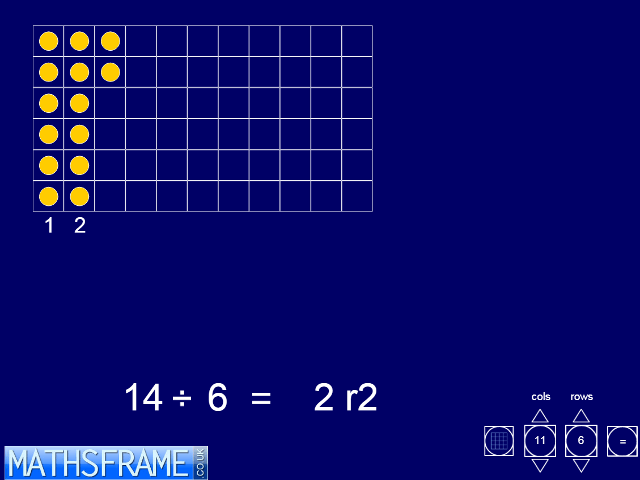## ITP Remainders after Division

I am remaking the ITPs so that they will work on all modern browsers and tablets. They will remain freely available to all without the need for a subscription.

This ITP sets up an empty grid into which you can place counters. Counters can be added singly, working from the first empty top left-hand corner cell, or in bulk clicking on a cell in the final row. As each column is completed it is numbered. The division calculation representing the number of highlighted counters divided by the number of rows can be revealed and hidden using the equals sign button. The answer appears when the equals sign in the calculation is clicked. Any remainder can also be shown as a fraction. In the example, below, there are 17 counters in a grid with 5 rows. Three columns are completed and there are 2 counters in the incomplete column. The displayed result will be:
17÷ 5 = 3r2 or 17÷ 5 = 3 2/5
Removing or highlighting extra counters will change the calculation displayed.
The ITP can be used to demonstrate division as grouping, and to develop children’s understanding of remainders and the notation for division. Keeping the number of counters fixed but changing the number of rows demonstrates the effect of changing the divisor in the calculation. Children can explore the effect different divisors has on the remainder and they can look for division calculations whose remainders satisfy particular properties.

For more multiplication and division resources click here.